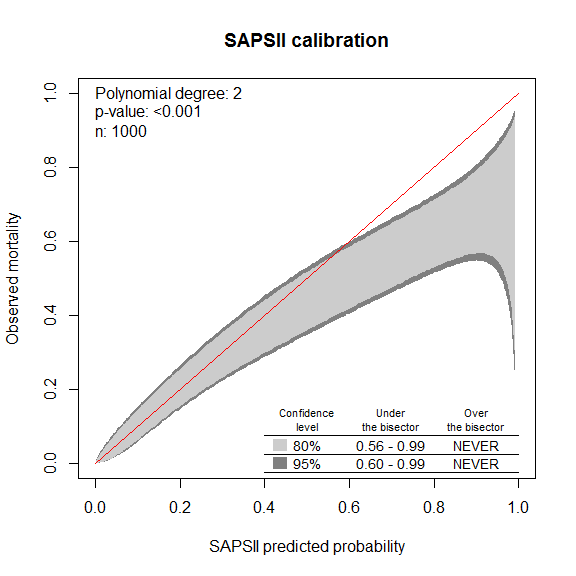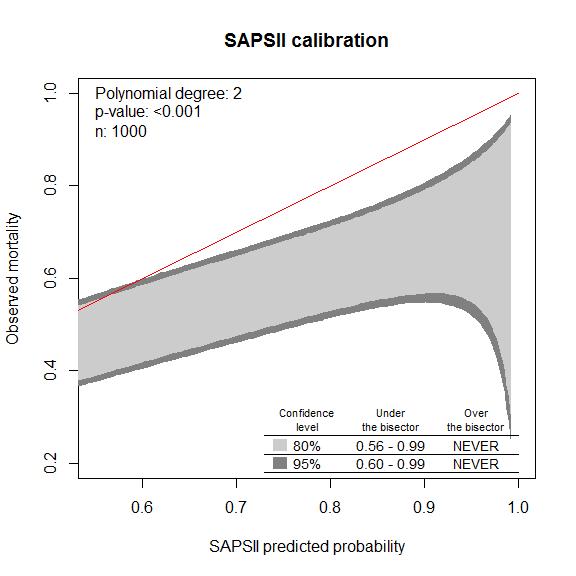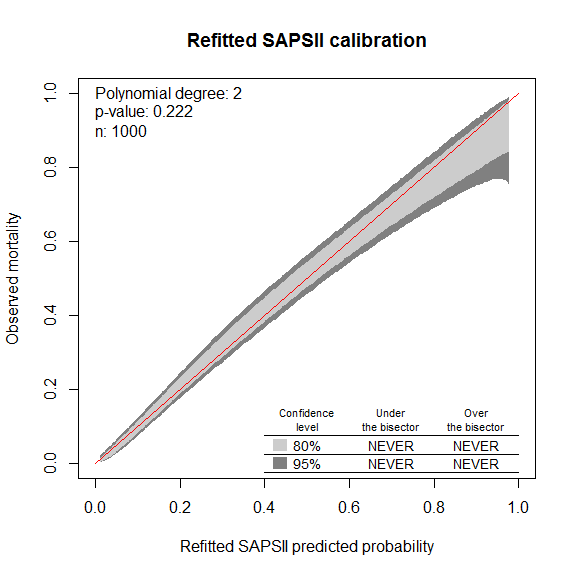## 1 Introduction

The package givitiR provides the functions to plot the GiViTI calibration belt and to compute the associated statistical test.

The name of the approach derives from the GiViTI (Gruppo Italiano per la valutazione degli interventi in Terapia Intensiva, Italian Group for the Evaluation of the Interventions in Intensive Care Units), an international network of intensive care units (ICU) established in Italy in 1992. The group counts more than 400 ICUs from 7 countries, with about the half of the participating centers continuosly collecting data on the admitted patients through the PROSAFE project (PROmoting patient SAFEty and quality improvement in critical care). For further information, see the GiViTI website (2016a) and the 2015 report of the PROSAFE project (2016b).

The GiViTI calibration belt and associated test apply to models estimating the probability of binary responses, such as logistic regression models. In particular, the approach implemented in the package is designed to evaluate the models’ calibration, that is, the capability of reliably estimating events rates. A model is well calibrated if the predicted probabilities accurately match the observed proportions of the response.

The calibration of a model can be evaluated in two settings: in independent samples or in the same dataset used to fit the model. In the former case the property is referred to as external calibration, in the latter as internal calibration (or goodness of fit). Most of the statistical methods evaluating the calibration apply to both the cases, even though each setting requires specific configurations of the methods. For example, the Hosmer-Lemeshow $$\hat{C}$$ statistic can be used in both the contexts, but the distribution under the null hypothesis is different. If the predictions are partitioned into $$g$$ groups, the null distribution of the $$\hat{C}$$ statistic is a $$\chi^2$$ with $$g-2$$ and $$g$$ degrees of freedom in the cases of the development dataset or of independent samples, respectively.

The approach implemented in this package is not an exception and it can be used both to externally validate a model and to evaluate the goodness of fit. However, the two cases have different requirements. When considering independent samples, the GiViTI calibration belt and the related test can be applied whatever is the method used to fit the model. Indeed, no assumption about the fit of the model is made in this case and the approach can be applied to any set of probabilities predicting the binary response. Conversely, the GiViTI calibration belt and test implemented in this package can be used on the development set only if the model considered is a logistic regression model. For more details, a full description of the methods is given by Finazzi et al. (2011), Nattino, Finazzi, and Bertolini (2014) and Nattino, Finazzi, and Bertolini (2016).

In this vignette we illustrate how the package givitiR can be used to construct the GiViTI calibration belt and test, with an application to clinical data. In particular, the examples are based on a dataset containing clinical information of 1,000 anonymous patients admitted to Italian intensive care units.

The rest of the document is organized as follows. Section 2 briefly describes the methodology, while Section 3 and 4 describe how to apply the GiViTI calibration belt approach to respectively evaluate a model on independent samples and to assess the goodness of fit on the development sample.

## 2 The GiViTI calibration belt and test

Consider a sample of size $$n$$, with $$o_1, ..., o_n$$ being the observed binary responses and $$e_1, ..., e_n$$ the predicted probabilities estimated with a logistic regression model. Hence, for each subject $$i$$, $$e_i$$ represents the estimate of $$P(o_i = 1)$$, i.e. the probability of the event of interest for that specific subject. The idea of the approach is to relate the predictions to the true probabilities of the event with a second logistic regression model, based on a polynomial transformation of the predictions. In particular, the procedure fits a polynomial logistic regression of the following form

$\mathrm{logit}(P(o_i = 1)) = \beta_0 + \beta_1 \mathrm{logit}(e_i) + \dots + \beta_m (\mathrm{logit}(e_i))^m,~~~~~~~~(1)$

where the logit function is defined as $$\mathrm{logit}(p) =\ln(p/(1-p))$$.

The degree $$m$$ of the polynomial is forwardly selected, on the basis of a sequence of likelihood ratio tests with significance level $$1-q$$. The starting model of the forward selection depends on the setting, that is, whether the calibration is evaluated on the development dataset or on independent samples. In the first case, the forward selection is started from a second order polynomial, since a first order polynomial would return estimates of the parameters $$\beta_0$$ and $$\beta_1$$ identically equal to 0 and 1 (see Nattino, Finazzi, and Bertolini (2016) for further details). In the case of external validation, the procedure is started from a first order polynomial. The purpose of these choices is to keep the model as simple as possible in each case, following the principle of parsimony in model building.

The proposed calibration test is a likelihood ratio test evaluating $$H_0$$: $$(\beta_0, \beta_1, \beta_2, ...,\beta_m) = (0,1,0,...,0)$$ versus the complementary hypothesis. Notably, the likelihood ratio statistic considered in the test is not $$\chi^2$$ distributed, since the forward selection affects the value of the statistic and, therefore, its null distribution (see Nattino, Finazzi, and Bertolini (2014) and Nattino, Finazzi, and Bertolini (2016)).

The GiViTI calibration belt consists in a graphical interpretation of Equation 1. By plugging the maximum likelihood estimates of the coefficients $$\beta_j$$ into the equation, it is possible to draw a curve, denoted as calibration curve, which represents a relationship between the predictions $$e_i$$ and the true probabilities $$P(o_i=1)$$. Then, for any confidence level $$1-\alpha$$, it is possible to derive a confidence band around the curve from the distribution of the statistical test. Such a confidence band is the calibration belt (see Nattino, Finazzi, and Bertolini (2014) and Nattino, Finazzi, and Bertolini (2016) for further details). The resulting band conveys the uncertainty in the estimated relationship between predictions and the probabilities of the true response, providing valuable information in the assessment of calibration.

The next sections show how to use the givitiR package to plot the GiViTI calibration belt and to compute the associated test.

## 3 Calibration assessment on independent samples

### 3.1 Data description

The examples consider a cohort of 1,000 anonymous patients admitted to Italian intensive care units (ICUs). The data have been collected within the PROSAFE project, an Italian observational study based on a continuous data collection of clinical data in more than 200 Italian ICUs. The purpose of the project is the continuous monitoring of the quality of care provided by the participating centers, on the basis of the outcome of the treated patients. The ongoing project is promoted by the GiViTI network (Gruppo Italiano per la valutazione degli interventi in Terapia Intensiva, Italian Group for the Evaluation of the Interventions in Intensive Care Units). The actual values of the variables have been modified to protect subject confidentiality.

The dataset icuData contains the information to apply the SAPSII model, a prognostic model developed to predict hospital mortality (Le Gall, Lemeshow, and Saulnier 1993). In particular, the model has been developed with logistic regression and it estimates the probability of death on the basis of 15 variables, describing patient’s demographics, comorbidities and clinical information. The model’s probability probSaps is a variable of the dataset. The dataset contains also the observed hospital survival of the patients. The GiViTI calibration belt and test are used to evaluate the calibration of the SAPSII on the dataset. Considering the “age” of the score (the model has been developed on data collected between the 1991 and 1992), it is legitimate to have concerns about the reliability of the model on recent data.

### 3.2 Plotting and interpreting GiViTI the calibration belt

The GiViTI calibration belt can be used to assess the calibration of the SAPSII model in the cohort. The function givitiCalibrationBelt implements the computations necessary to produce the calibration belt plot. Since this dataset is not the development dataset, the procedure for the assessment of external validation must be applied. This is done by defining devel = "external". Remember that the GiViTI calibration belt can be constructed on independent samples whatever is the method used to fit the model. Therefore, we could have applied the calibration belt to the SAPSII probabilities even if the model were fitted on a method different from logistic regression.

The method plot applied to an object of type givitiCalibrationBelt (as produced by the function givitiCalibrationBelt) generates the plot.

library(givitiR)
data("icuData")
cb <- givitiCalibrationBelt(o = icuData$outcome, e = icuData$probSaps,
devel = "external")
plot(cb, main = "SAPSII calibration",
xlab = "SAPSII predicted probability",
ylab = "Observed mortality")## $m ##  2 ## ##$p.value
##  6.269563e-10

By default, the 80%- and 95%-confidence level calibration belt are plotted, in light and dark grey respectively. Different confidence levels can be considered with the confLevels argument– for example, confLevels = .95 produces a 95%-confidence level calibration belt only, confLevels = c(.90,.95) produces a 90%- and 95%-confidence level calibration belt.

The table in the bottom-right side of the figure reports the ranges of the predicted probabilities where the belt significantly deviates from the bisector. Notably, the calibration belt contains the bisector (representing the identity between predicted probability and observed response rate) for the predictions in the middle-low range. Hence, the SAPSII predictions match the average observed rates in the middle-to-low range.

Conversely, the calibration belt is under the bisector for probabilities higher than 0.56 or 0.60 (using respectively a confidence levels of 80% and 95%). This provides evidence that the SAPSII model significantly overestimates the mortality for high risk patients– if the belt is below the bisector, the predictions are larger than the actual observed rates of the event.

The progresses in medicine during the last decades may be an explanation of this result. The mortality of ICU patients has surely decreased since the time of SAPSII development, and it is plausible that the most severe patients have benefited of greater reductions in the risk of dying. This could be the reason of the important overestimation of the score for the high risk patients.

### 3.3 Graphical parameters

The overall calibration of the model is synthesized into the test’s p-value, which is reported in the top-left corner of the figure. In addition, the sample size $$n$$ and the polynomial order m of the calibration curve (see Section 2) are reported in the plot. The printing of these details in the graphical area can be suppressed setting as FALSE the arguments pvalueString, nString and polynomialString in the function plot. Analogously, the printing of the table in the bottom-right corner can be suppressed setting the argument table to FALSE.

It is possible to obtain plots zooming possible ranges of interest by modifying the plotted x and y limits in the vectors xlim and ylim.

plot(cb, main = "SAPSII calibration",
xlab = "SAPSII predicted probability",
ylab = "Observed mortality",
xlim = c(0.55,1), ylim = c(0.2,1))## $m ##  2 ## ##$p.value
##  6.269563e-10

The number of points defining the edges of the belt can be modified with the nPoints argument of givitiCalibrationBelt (the default value is 200). Reducing the number of points can substantially speed up the production of the plot in large datasets. However, this number also affects the estimate of the probabilities where the belt crosses the bisector (i.e. the limits of the intervals reported in the table): the greater the value of nPoints, the higher the precision in the estimate of these values. If the production of the belt is too slow but the analysis requires an iterative construction of many belts– for example in exploratory analyses– a possible strategy is to decrease the value of nPoints to values much smaller than the default (say 20 or 50), taking into account the possible larger uncertainty in the interpretation of the plots. Finally, when the analysis is set up, the number of points can be increased to the default value to achieve more accurate estimates of the potential deviations from the bisector.

The parameter maxDeg allows the user to modify the maximum degree that can potentially be reached with the forward selection process (see Section 2). The threshold value thres corresponds to the value of $$q$$, i.e. 1 minus the significance of the iterative sequence of likelihood ratio tests in the forward selection. Extensive simulations evaluating the sensitivity of the GiViTI calibration belt and test to the choice of these parameters have been carried out in Nattino, Finazzi, and Bertolini (2014). Our suggestion is to keep the default values of m=4 and thres=.95.

The function givitiCalibrationTest implements the statistical test only, without the production of the plot. The value of the statistic, the p-value (also reported in the calibration belt plot) and the estimates of the parameters $$\beta_j$$ for $$j$$ from 0 to $$m$$ are reported in the output.

givitiCalibrationTest(o = icuData$outcome, e = icuData$probSaps,
devel = "external")
##
##  GiViTI calibration test - external validation
##
## data:  e = 'Predictions' and o = 'Binary outcome'
## Stat = 51.27, p-value = 6.27e-10
## alternative hypothesis: two.sided
## null values:
## beta0 beta1 beta2
##     0     1     0
## sample estimates:
##       beta0       beta1       beta2
## -0.25342187  0.67880695 -0.09505753

## 4 Calibration assessment on the development sample

### 4.1 Plotting and interpreting the GiViTI calibration belt

The conclusion of the first step is that the SAPSII model significantly overestimates the probability of death for high-risk patients. It is plausible that the progresses in medicine over the last 20 years have improved the outcome of patients, in particular for the most severe. This is a possible explanation of the overestimation of the risk.

A possible way to address the requirement of a reliable prognostic model on the icuData sample is to fit a new model on the data. Since the clinical information needed to construct the SAPSII score were available in the dataset, we can think of fitting a new logistic regression model using the predictors of the SAPSII model as covariates. Of course, the good performance of the model on the development sample is not sufficient to infer the generalizability of the model, which would require external validations. However, good performance within the development sample may be sufficient if the prognostic model is not needed to be exported, for example if the model is going to be used as a confounder in a multivariable analysis or as a benchmark in the assessment of quality of different centers.

Therefore, suppose to refit the SAPSII model. Here we are not focusing on the steps of model development, we are just considering the calibration of the refitted SAPSII using all the original predictors of the score. This is only one of the possible models that can be built on this sample. In the following code, we are fitting the model on the icuData and computing the predicted probabilities probRefittedSaps

formulaSAPS <- formula(outcome ~ relevel(adm,'schSurg') + relevel(chronic,'noChronDis') +
relevel(age,'<40') + relevel(gcs,'14-15') +
relevel(BP,'100-199') + relevel(HR,'70-119') +
relevel(temp,'<39') + relevel(urine,'>=1') +
relevel(urea,'<0.60') + relevel(WBC,'1-19') +
relevel(potassium,'3-4.9') + relevel(sodium,'125-144') +
relevel(HCO3,'>=20') + relevel(bili,'<4') +
relevel(paFiIfVent,'noVent') )

refittedSaps <- glm(formula = formulaSAPS, family=binomial(link='logit'),
na.action = na.exclude, data = icuData)

icuData$probRefittedSaps <- predict(refittedSaps, type = "response") The evaluation of the calibration on the development dataset proceeds similarly to the case of external validation. Note that we are allowed to apply the GiViTI calibration belt and the associated test to evaluate the fitted model only because such a model has been fitted with logistic regression. The same functions can be used to generate and plot the calibration belt. The only difference is the definition of the argument devel="internal", which specifies the use of the method for internal validation. cbInternal <- givitiCalibrationBelt(o = icuData$outcome, icuData$probRefittedSaps, devel = "internal") plot(cbInternal, main = "Refitted SAPSII calibration", xlab = "Refitted SAPSII predicted probability", ylab = "Observed mortality")##$m
##  2
##
## $p.value ##  0.2215862 The interpretation of the output is analogous to the one of the external validation sample. In this case, no evidence of lack of calibration emerges from the calibration belt, which encompasses the bisector in the whole 0-1 range. Accordingly, the p-value of 0.22 suggests that the calibration of the model on the development sample is acceptable. The refitted SAPSII can therefore be considered as well calibrated on this cohort. ### 4.2 Graphical parameters and other utilities The graphical parameters and options are the same discussed in the previous section for external calibration. It is also possible to apply the calibration test on the model, specifying devel = "internal" as in the function that produces the GiViTI calibration belt. givitiCalibrationTest(o = icuData$outcome, e = icuData\$probRefittedSaps,
devel = "internal")
##
##  GiViTI calibration test - internal validation
##
## data:  e = 'Predictions' and o = 'Binary outcome'
## Stat = 1.4941, p-value = 0.2216
## alternative hypothesis: two.sided
## null values:
## beta0 beta1 beta2
##     0     1     0
## sample estimates:
##      beta0      beta1      beta2
##  0.0391975  0.9184636 -0.0527476